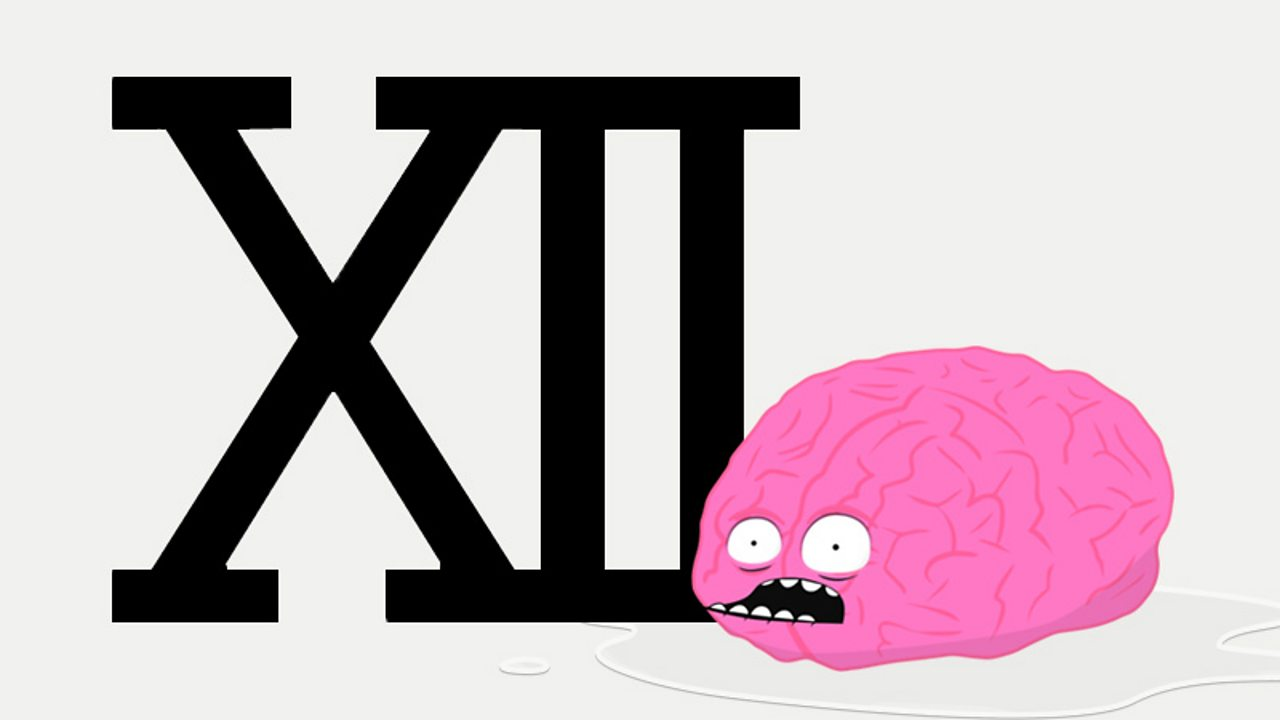# What is analogue and digital time?

There are 24 hours in one day, but the day can be measured by splitting it into two halves. The first 12 hours of the day - from midnight to midday - are called AM, and the next twelve hours are called PM.

## Roman numerals

The Romans used a different numbering system from the one that we use today. Their numbers were shown by letters.

I = 1, V = 5, X = 10, L = 50 and C = 100

### Here are some examples

V is 5, so VI is 5 + 1 or 6.

IV is 4. When the I is before the V it means 1 less than 5.X is 10, so XII is 10 + 2, or 12